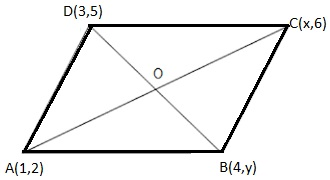Newbie

# If (1, 2), (4, y), (x, 6) and (3, 5) are the vertices of a parallelogram taken in order, find x and y. Q.6

• 0

Give me the best solution of coordinate geometry of ncert class 10th , please help me how i can solve this question easily and quickly .If (1, 2), (4, y), (x, 6) and (3, 5) are the vertices of a parallelogram taken in order, find x and y.

Share

1. Let A,B,C and D be the points of a parallelogram : A(1, 2), B(4, y), C(x, 6) and D(3, 5).Since the diagonals of a parallelogram bisect each other, the midpoint is same.

To find the value of x and y, solve for midpoint first.

Midpoint of AC = ( (1+x)/2 , (2+6)/2 ) = ((1+x)/2 , 4)

Midpoint of BD = ((4+3)/2 , (5+y)/2 ) = (7/2 , (5+y)/2)

Midpoint of AC and BD are same, this implies

(1+x)/2 = 7/2 and 4 = (5+y)/2

+ 1 = 7 and 5 + = 8

= 6 and = 3

• 0